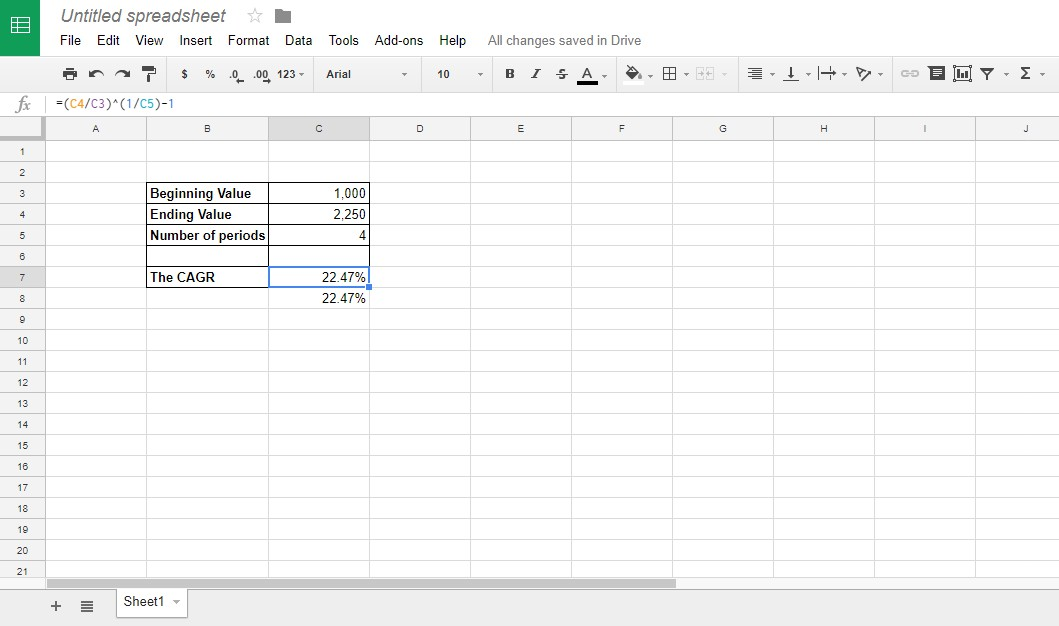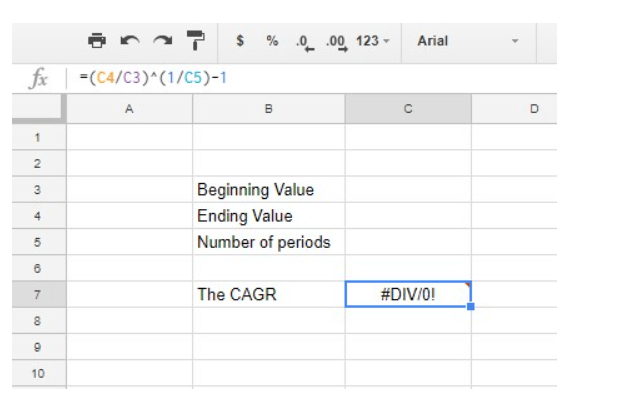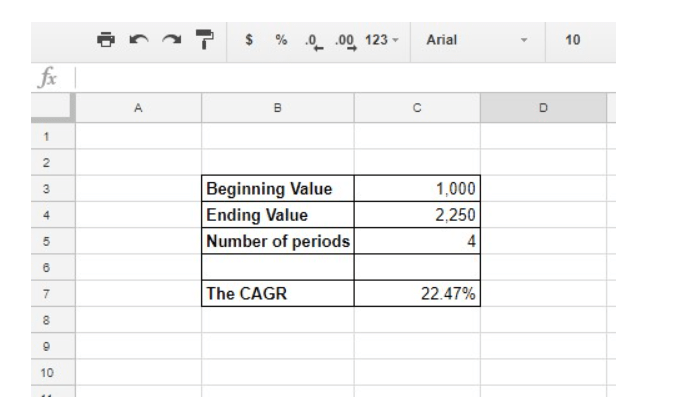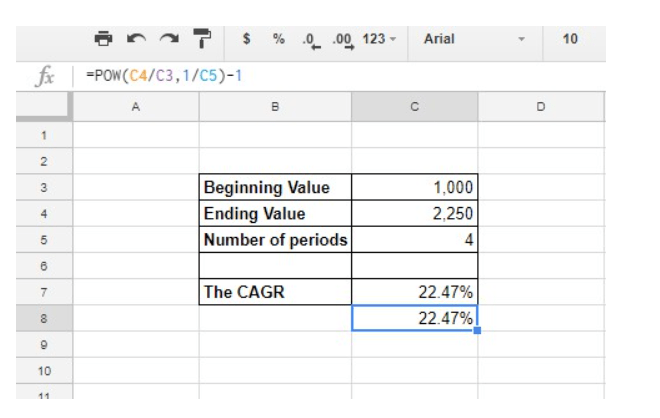Lots of businesspeople use Google Sheets as a web-based application to do financial calculations, and lots of people use it to handle their personal finances as well, because the cloud-based spreadsheet app includes many powerful financial functions and can be used to set up a wide variety of investment spreadsheets. The compound annual growth rate, otherwise known as CAGR, is one of the handy formulas Sheets users can add to their spreadsheets with or without the application’s functions. With the CAGR formula, you can set up a spreadsheet that shows the average annual growth rate, in percentage terms, for a series of figures over multiple time periods.## The CAGR Formula

Investment analysts and business owners frequently refer to something known as “CAGR” when discussing projects or proposals. CAGR stands for “compound annual growth rate”, and this formula is widely used for investment analysis. Spreadsheets often include tables for a series of values that show you the year-to-year growth rate for each figure. For example, an investment might grow by 23%, 11% and 13% in three separate years. However, what would the average annual percentage increase be for those years?

You could find an average percentage increase for those values by adding 23%, 11% and 13% together and then dividing them by three. That would amount to an average annual growth of 15.66%. However, that does not factor in the compounding effects of investments. CAGR is an average that also takes compounding into account; the CAGR gives you the growth rate that, if it had been the growth rate in each successive year, would produce the current total from the starting value. In effect, it is the real average rate of annual return for an investment.

CAGR is not a built-in formula in Google Sheets, and so to use it in your spreadsheets, you will need to have Sheets do the computation directly. Fortunately, the CAGR formula is not complex.

The CAGR formula is: EV / BV ^ (1/n) – 1. EV and BV are the ending and beginning values, while n is the number of time periods (usually months or years) for which you are calculating an average. The ^ character means “to the power of”; we take the ratio of EV / BV and raise it to the power of 1/n. Subtracting one (one = 100%)

Before we add the CAGR formula to a spreadsheet, check out this calculator that returns the compound average growth rate for numbers entered. On that page, you enter the starting and ending values along with the number of periods. You can set up exactly the same calculator in Sheets, and then use the online calculator to make sure your spreadsheet version is properly configured.

1. To start, open a blank spreadsheet in Sheets and enter ‘Beginning Value‘ in cell B3 of the spreadsheet, ‘Ending Value’ in B4, ‘Number of periods‘ in cell B5, and ‘The CAGR‘ in cell B7.2. Now, in cell C7, click within the fx bar, enter ‘=(C4/C3) ^ (1/2)-1‘ and press Enter.3. Now enter ‘1,000‘ in cell C3, ‘2,250‘ in C4, ‘4‘ in cell C5 for the number of time periods.4. Cell C7 will return the value 0.2247448714. To convert that cell to a percentage, select C7 and press the Format as percentage button. Then the compound annual growth rate value in C7 will display as 22.47% as shown directly below.5. As a final touch, add some formatting to the compound annual growth rate calculator. Select the cell range B3:C7 with cursor and press the Borders button on the toolbar. Select to add borders to all cells in the calculator as below.6. Select the cells B3:B7 by dragging the cursor over them. Press the Bold button on the toolbar. That option adds bold text formatting to the headings.7. You can add colors to the calculator’s cells. Select the cells to format and click the Fill color toolbar option. That opens a palette from which you can choose new colors.

## The POW Function

Another way to calculate the CAGR is to use Sheets’ POW function. The syntax for that function is: POW (base, exponent). For the CAGR formula, the base is the end value / beginning value and the exponent is 1/n.

1. Select cell C8 in your spreadsheet, enter ‘=POW(C4/C3,1/C5)-1‘ in the fx bar, and press Enter. Cell C8 will include the CAGR value of 0.2247448714. Convert the C8 formatting to percentage as you did for C7.As you can see, the POW function cell now includes exactly the same common annual grow rate figure as C7. These are just two different ways to get the same value, you can use whichever one you feel more comfortable with.

That’s how you can set up a CAGR calculator in Sheets with the annual growth rate formula and POW function. RATE and IRR are also two other functions that you can find the compound annual growth rate with. If interested, you can also add the CAGR formula to Excel spreadsheets.Ian says:
Thanks for this. I really needed this exact content.